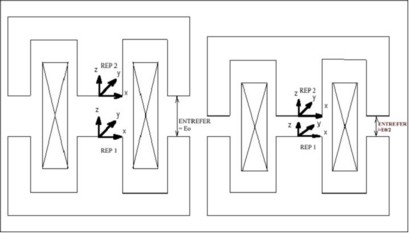# Parameterization

## Introduction

The parameterization of the geometry is one of the strong points of the geometry building module.

It is possible to parameterize:

• dimensions of workpieces
• relative displacements of pieces (variable air-gap…).

## Parameterization tools

A geometrical object can be parameterized:

• on one hand, using the geometrical parameters,
• on the other hand, using the local coordinate systems (coordinate systems defined with respect to a reference coordinate system).

These concepts are presented in the example below.

## Example

The example refers to the study of a contactor, concerning the force acting on the moving part for various values of the air-gap.

The fixed part is defined in a local coordinate system REP1 of center (0, 0, 0), and the moving part in a local coordinate system REP2 of center (0, 0, AIR-GAP). AIR-GAP is a parameter whose value is equal to the air-gap thickness.

To study various positions of the moving part, and thus various values of the air-gap, it is enough to modify the value of the corresponding parameter (AIR-GAP) and to treat the corresponding case.## Principle and limits

Each time that a geometrical entity is modified, all the entities depending on this geometrical entity are automatically reevaluated through the database tools.

Modifying a parameter or a coordinate system entails the modification of the points, then of the lines, and then of the faces and volumes that are attached to this parameter.

Important: the coherence of the topology (intersection, superposition…) is not verified by the software. This verification is a user task.

In the previous example, a null value of the AIR-GAP parameter leads to a modification of the geometry topology that can not be realized due to superposed points and lines. This limit case cannot be treated by parameterization.